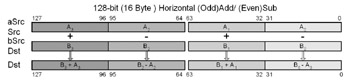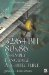Special -- FP Vector Addition and SubtractionSpecial FP Vector Addition and Subtraction

The addition and subtraction of simultaneous vectors are a relatively simple matter for vector math instructions to handle. SSE3 added simultaneous functionality, while older versions have to simulate it.

Vector Floating-Point Addition and Subtractiond (odd) = b (odd) + a (odd) d (even) = b (even) a (even)

d (3) = b (3) + a (3) d (2) = b (2) a (2) d (1) = b (1) + a (1) d (0) = b (0) a (0)

Mnemonic

P

PII

K6

3D!

3Mx+

SSE

SSE2

A64

SSE3

E64T32/64-Bit 80x86 Assembly Language Architecture
ISBN: 1598220020
EAN: 2147483647
Year: 2003
Pages: 191

Similar book on Amazon